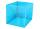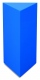# Right triangle - 6th grade (11y) - examples

1. It is rectangular?Size of two of the angles in a triangle are: α=110°, β=40°. Is it a right triangle?
2. SimilarityAre two right triangles similar to each other if the first one has a acute angle 70° and second one has acute angle 20°?
3. TriangleCan be rectangular triangle equilateral?
4. TaskI have homework. The cube has an edge 7 cm long and I must find wall and body diagonal.
5. Triangle P2Can triangle have two right angles?
6. Area of RT 2Calculate the area of right triangle whose legs have a length 5.8 cm and 5.8 cm.
7. Right triangleRight triangle legs has lengths 630 mm and 411 dm. Calculate the area of this triangle.
8. Perpendicular prismCalculate the volume of the perpendicular prism if its height is 60.8 cm and the base is a rectangular triangle with 40.4 cm and 43 cm legs.

We apologize, but in this category are not a lot of examples.
Do you have an interesting mathematical example that you can't solve it? Enter it, and we can try to solve it.

To this e-mail address, we will reply solution; solved examples are also published here. Please enter e-mail correctly and check whether you don't have a full mailbox.

See also our right triangle calculator.